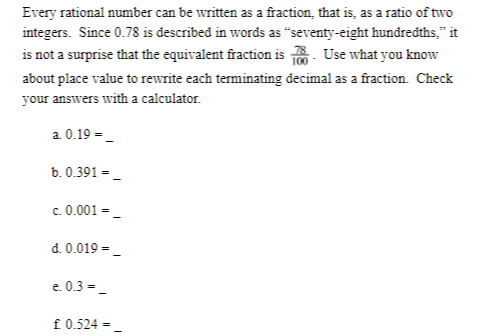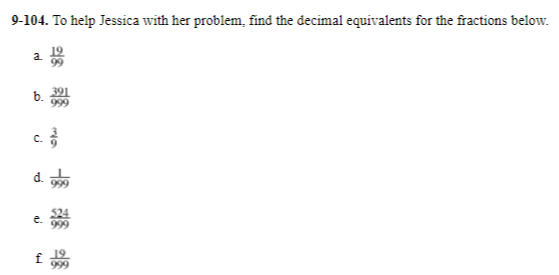What kind of number is it? (part 2)
starstarstarstarstarstarstarstarstarstar
by Lonnie Myers
| 8 Questions
Note from the author:
fractions for terminating and repeating decimals
1
1

1. What do you notice about the decimal forms of rational numbers compared to irrational numbers?
2
1
1. Is
3
1
Every rational number can be written as a fraction, that is, as a ratio of two integers.  Since 0.78 is described in words as “seventy-eight hundredths,” it is not a surprise that the equivalent fraction is 78/100.  Use what you know about place value to rewrite each terminating decimal as a fraction.  Check your answers with a calculator.4
1
Jessica knows that .57575757 is a rational number, so she should be able to write it as a fraction.  She wonders how to rewrite it, though.  She started to rewrite it as 57/100 but she is not sure if that correct.  Is 57/100 equal to .57575757?  Explain your answer.
5
1
To help Jessica with her problem, find the decimal equivalents for the fractions below. Enter answers with 6 numbers to the right of the decimal point followed with 3 dots. Example .272727...6
1
What patterns do you see between the fractions and their equivalent decimals? What connections do these fractions have with those you found in problem 3? Be ready to share your observations.

7
1
Use your pattern to predict the fraction equivalent for 0.242424...  Then test your guess with a calculator.
8
1